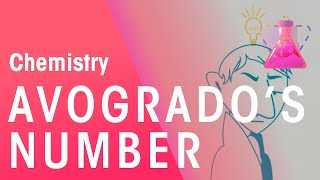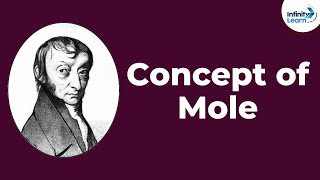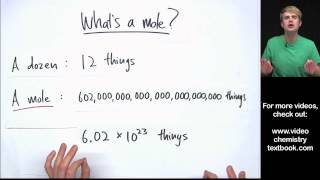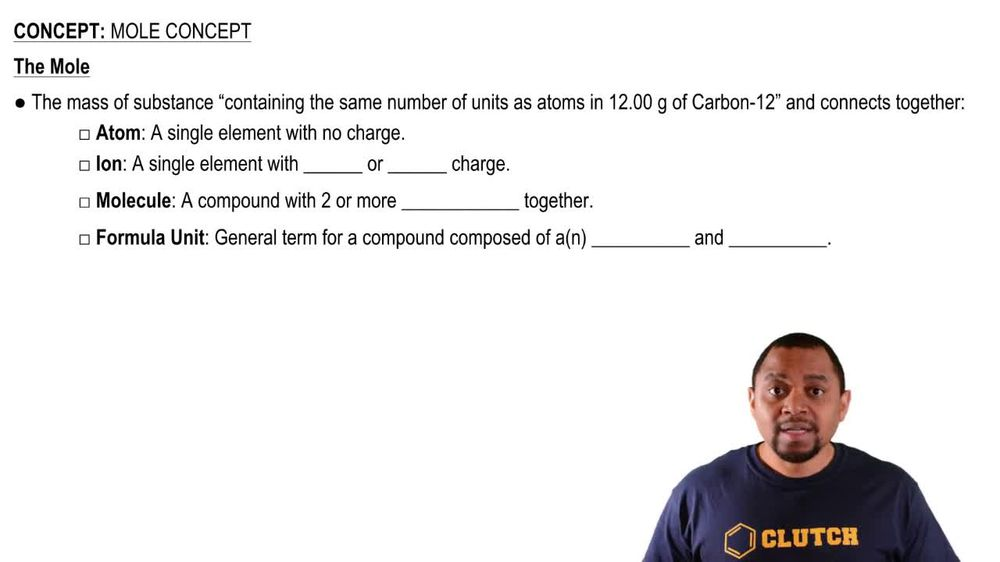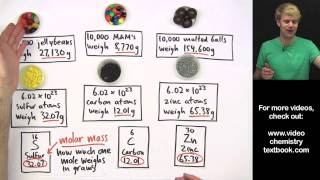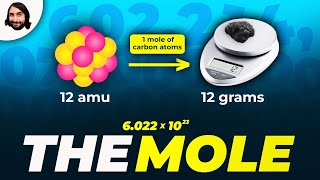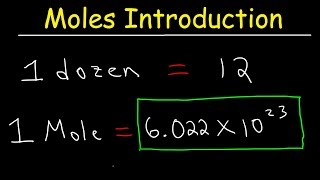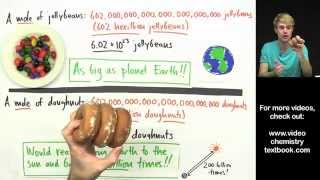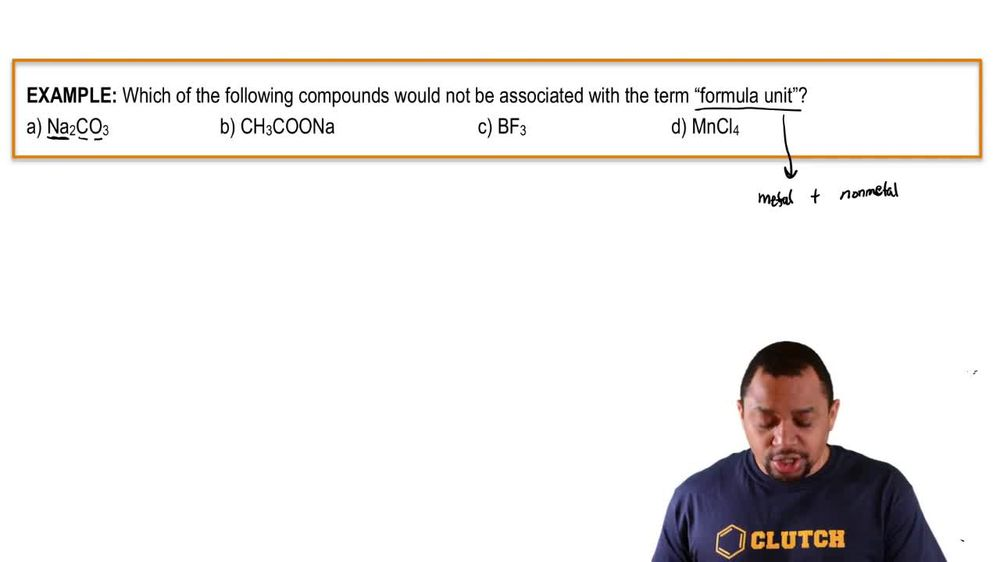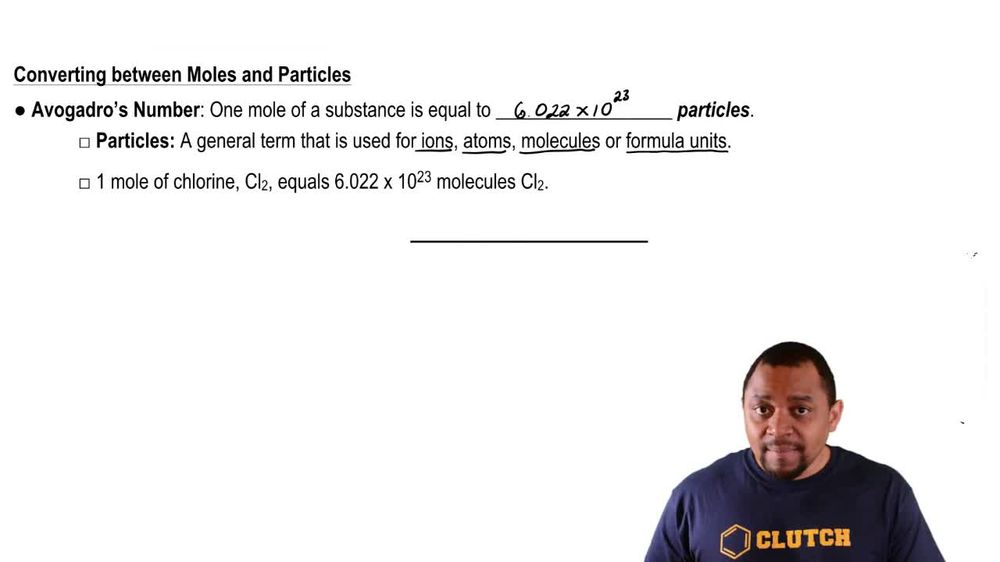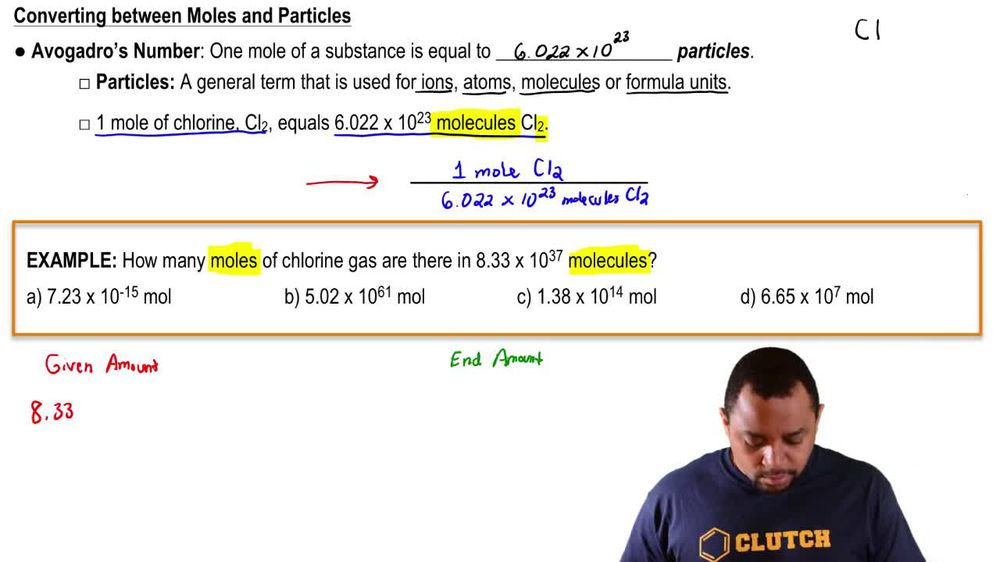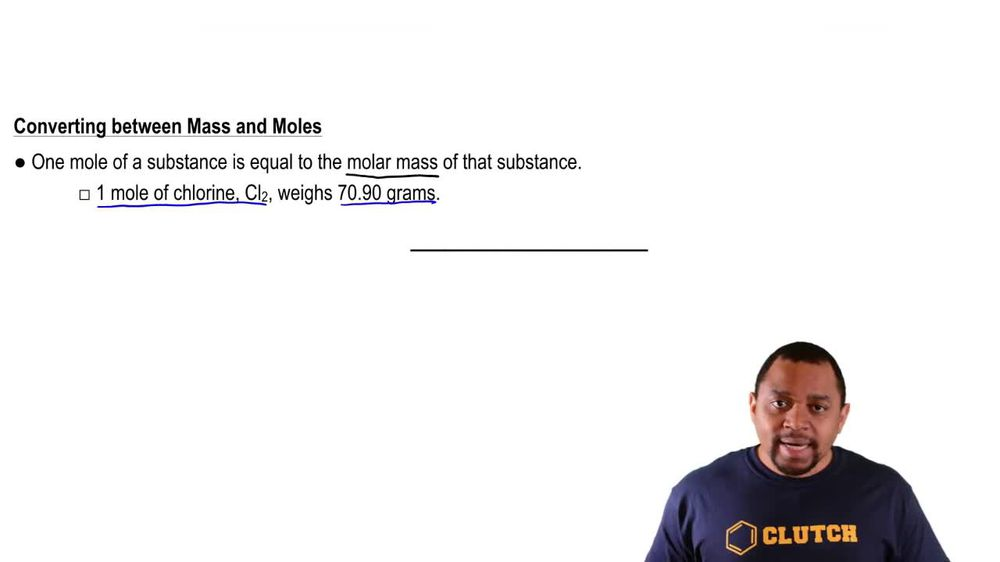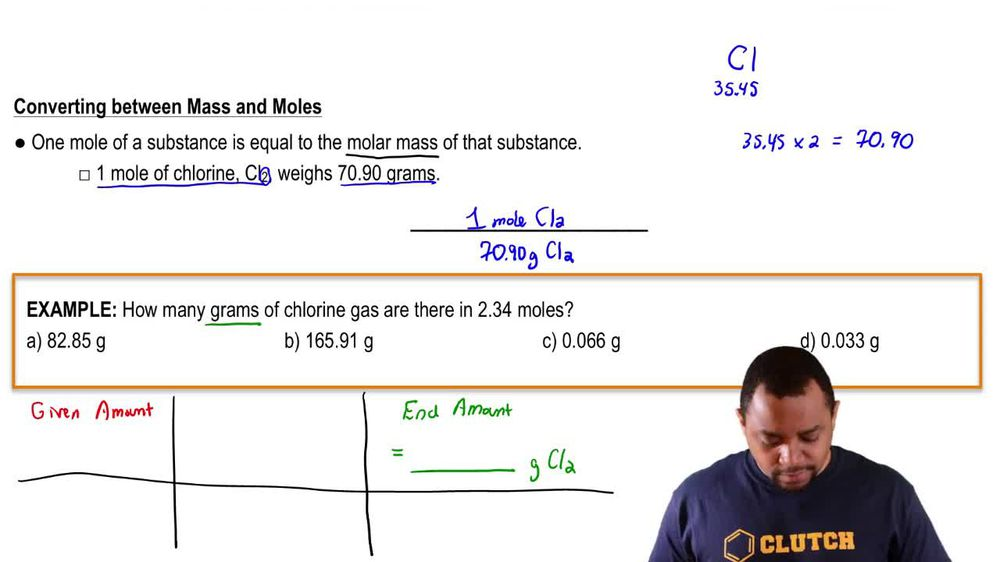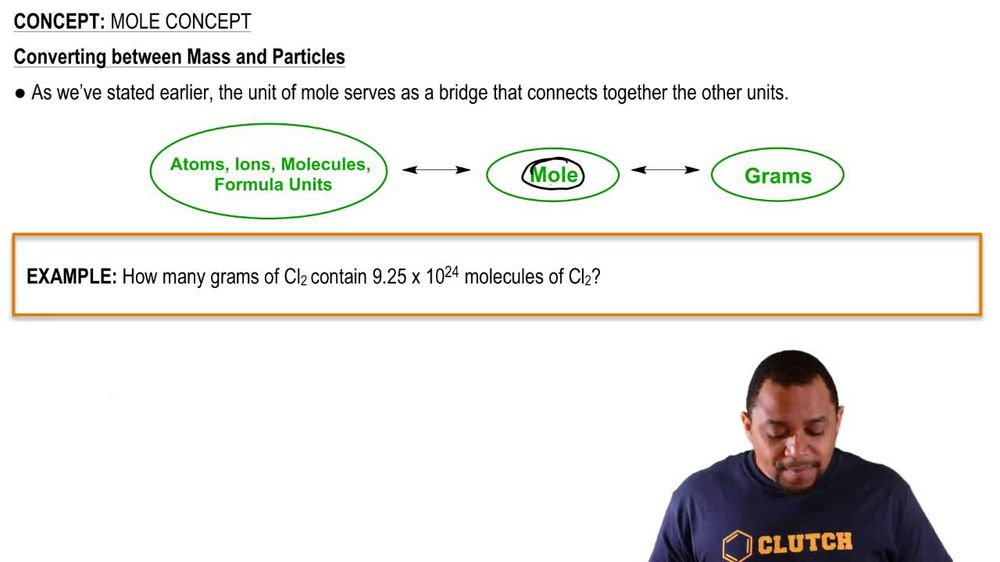Start typing, then use the up and down arrows to select an option from the list.
1. 2. Atoms & Elements2. Mole Concept
Problem

# (b) The mole is a unit used to represent a very large number of atoms. How many atoms are equivalent to 1 mol of atoms?

Relevant Solution2m
Play a video:
Hi everyone for this problem it reads identify if the following statement is true or false. One mole of molecules is equivalent to 6.22 times 10 to the molecules. Okay, so we want to identify whether or not this is true or false. This number here is something we should recognize as avocados number. Okay, and avocados number Is defined as 6.02, 2 times 10 to the 23rd entities per one mall of entities. Okay, what does that mean? Entities by entities? This can mean Adams, ions, molecules, particles and formula units. Okay, so this is the definition of avocados number. And the question is asking us one mole of molecules is equivalent to 6.22 times 10 to the 23rd molecules. So we see here that are entity is molecules and because that's what's written here, one mole of molecules is equivalent to 6.22 times 10 to the 23rd molecules. So we see here that avocados number is for every one mole of entities. There are 6.22 times 10 to the 23rd of the same entity. So with that, that makes this statement true. Okay, so this is true. Based off of the definition for avocados number, which we just reviewed. Okay, so that's it for this problem. I hope this was helpful